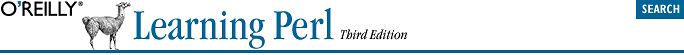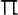home | O'Reilly's CD bookshelfs | FreeBSD | Linux | Cisco | Cisco ExamThis appendix contains the answers to the excerses that appear throughout the book.

## A.1. Answers to Chapter 2 Exercises

1. Here's one way to do it:

```#!/usr/bin/perl -w
\$pi = 3.141592654;
\$circ = 2 * \$pi * 12.5;
print "The circumference of a circle of radius 12.5 is \$circ.\n";```

As you see, we started this program with a typical #! line; your path to Perl may vary. We also turned on warnings.

The first real line of code sets the value of \$pi to our value of. There are several reasons a good programmer will prefer to use a constant value like this: it takes time to type 3.141592654 into your program if you ever need it more than once. It may be a mathematical bug if you accidentally used 3.141592654 in one place and 3.14159 in another. There's only one line to check on to make sure you didn't accidentally type 3.141952654 and send your space probe to the wrong planet. It's easier to type \$pi than, especially if you don't have Unicode. And it will be easy to maintain the program in case the value ofever changes.

If you'd prefer a more formal sort of constants, the constant pragma may be what you're looking for.

It nearly did change by a legislative act in the state of Indiana. http://www.urbanlegends.com/legal/pi_indiana.html

Next we calculate the circumference, storing it into \$circ, and we print it out in a nice message. The message ends with a newline character, because every line of a good program's output should end with a newline. Without it, you might end up with output looking something like this, depending upon your shell's prompt:

```The circumference of a circle of radius 12.5 is
78.53981635.bash-2.01\$&cursor;```

The box represents the input cursor, blinking at the end of the line, and that's the shell's prompt at the end of the message. Since the circumference isn't really 78.53981635.bash-2.01\$, this should probably be construed as a bug. So use \n at the end of each line of output.

We asked O'Reilly to spend the extra money to print the input cursor with blinking ink, but they wouldn't do it for us.

2. Here's one way to do it:

```#!/usr/bin/perl -w
\$pi = 3.141592654;
print "What is the radius? ";
\$circ = 2 * \$pi * \$radius;

This is just like the last one, except that now we ask the user for the radius, and then we use \$radius in every place where we previously used the hard-coded value 12.5. If we had written the first program with more foresight, in fact, we would have had a variable named \$radius in that one as well. Note that we chomped the line of input. If we hadn't, the mathematical formula would still have worked, because a string like "12.5\n" is converted to the number 12.5 without any problem. But when we print out the message, it would look like this:

```The circumference of a circle of radius 12.5
is 78.53981635.```

Notice that the newline character is still in \$radius, even though we've used that variable as a number. Since we had a space between \$radius and the word "is" in the print statement, there's a space at the beginning of the second line of output. The moral of the story is: chomp your input unless you have a reason not to do that.

3. Here's one way to do it:

```#!/usr/bin/perl -w
\$pi = 3.141592654;
print "What is the radius? ";
\$circ = 2 * \$pi * \$radius;
\$circ = 0;
}

Here we added the check for a bogus radius. Even if the given radius was impossible, the returned circumference will at least be nonnegative. You could have changed the given radius to be zero, and then calculated the circumference, too; there's more than one way to do it. In fact, that's the Perl motto: There Is More Than One Way To Do It. And that's why each exercise answer starts with "Here's one way to do it."

4. Here's one way to do it:

```print "Enter first number: ";
chomp(\$one = <STDIN>);
print "Enter second number: ";
chomp(\$two = <STDIN>);
\$result = \$one * \$two;
print "The result is \$result.\n";```

Notice that we've left off the #! line for this answer. In fact, from here on, we'll assume that you know it's there, so you don't need to read it each time.

Perhaps those are poor choices for variable names. In a large program, a maintenance programmer might think that \$two should have the value of 2. In this short program, it probably doesn't matter, but in a large one we could have called them something more descriptive, with names like \$first_response.

In this program, it wouldn't make any difference if we forgot to chomp the two variables \$one and \$two, since we never use them as strings once they've been set. But if next week our maintenance programmer edits the program to print a message like: The result of multiplying \$one by \$two is \$result.\n, those pesky newlines will come back to haunt us. Once again, chomp unless you have a reason not to chomp -- like in the next exercise.

Chomping is like chewing -- not always needed, but most of the time it doesn't hurt.

5. Here's one way to do it:

```print "Enter a string: ";
\$str = <STDIN>;
print "Enter a number of times: ";
chomp(\$num = <STDIN>);
\$result = \$str x \$num;
print "The result is:\n\$result";```

This program is almost the same as the last one, in a sense. We're "multiplying" a string by a number of times. So we've kept the structure of the previous exercise. In this case, though, we didn't want to chomp the first input item -- the string -- because the exercise asked for the strings to appear on separate lines. So, if the user entered fred and a newline for the string, and 3 for the number, we'd get a newline after each fred just as we wanted.

In the print statement at the end, we put the newline before \$result because we wanted to have the first fred, printed on a line of its own. That is, we didn't want output like this, with only two of the three freds aligned in a column:

```The result is: fred
fred
fred```

At the same time, we didn't need to put another newline at the end of the print output because \$result should already end with a newline.

In most cases, Perl won't mind where you put spaces in your program; you can put in spaces or leave them out. But it's important not to accidentally spell the wrong thing! If the x runs up against the preceding variable name \$str, Perl will see \$strx, which won't work.